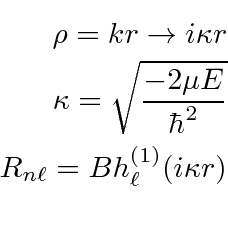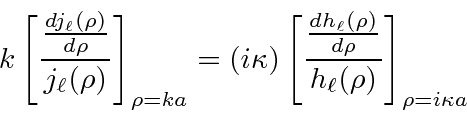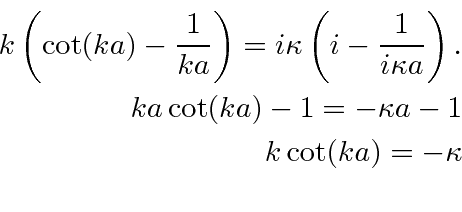## Bound States in a Spherical Potential Well *

We now wish to find the energy eigenstates for a spherical potential well of radiusand potential.We must use the Bessel function near the origin.We must use the Hankel function of the first type for large.To solve the problem, we have to match the solutions at the boundary. First match the wavefunction.Then match the first derivative.We can divide the two equations to eliminate the constants to get a condition on the energies.This is often called matching the logarithmic derivative.

Often, theterm will be sufficient to describe scattering. For, the boundary condition isDividing and substituting for, we getThis is the same transcendental equation that we had for the odd solution in one dimension.The number of solutions depends on the depth and radius of the well. There can even be no solution.Jim Branson 2013-04-22